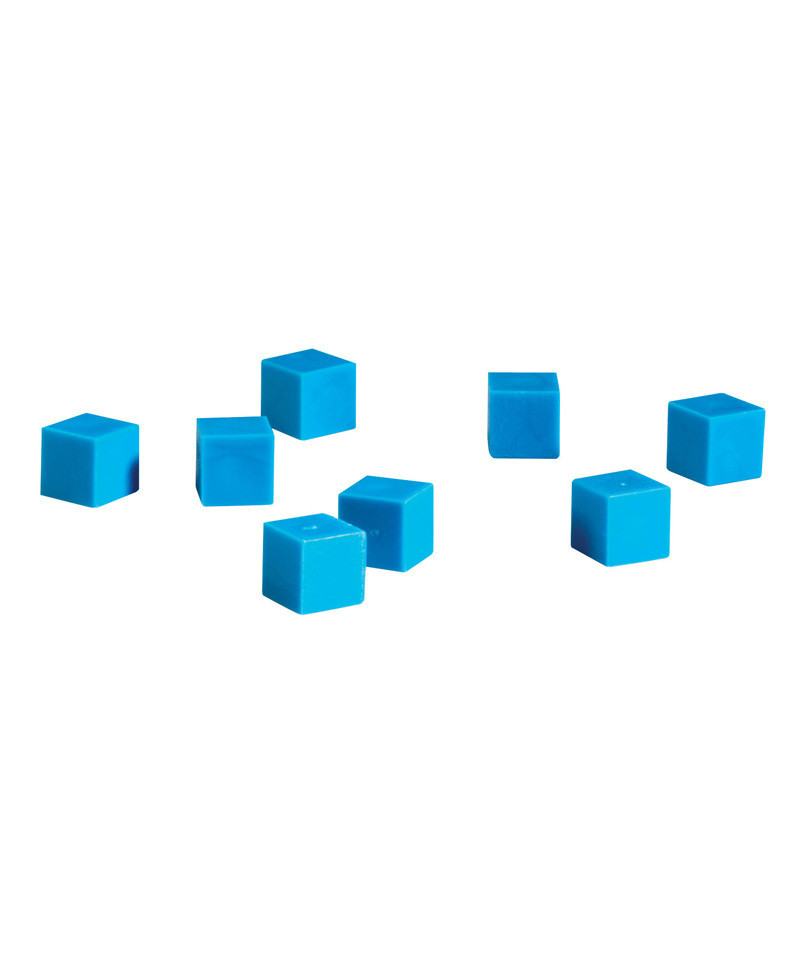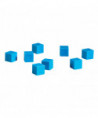•# (6 EA) BASE TEN UNITS PLASTIC BLUE

\$20.94
Tax excluded
Quantity
Use these Plastic Base Ten manipulatives to engage students in concrete demonstrations of place value and base ten concepts. Each cube measures one square centimeter. Set of 100 cubes each, bundle of 6, 600 cubes total

Use these Plastic Base Ten manipulatives to engage students in concrete demonstrations of place value and base ten concepts. Each cube measures one square centimeter. Set of 100 cubes each, bundle of 6, 600 cubes total
LER0924BN
14 Items